Main Content

# Loading Using Lumped Elements

This example shows how to load an antenna using a lumpedElement. You can load an antenna to make it smaller, enable matching at the feedline, or to make antennas larger to obtain higher performance. The example below demonstrates creating a simple matching network by adding a lumped load at the feed.

### Define a lumpedElement

The lumpedElement allows the user to specify a complex load. The load can be frequency independent (scalar) or dependent (vector). You can define the frequency variation as a vector using the Frequency property. You can also choose the location on the antenna surface where the load needs to be specified. To perform impedance matching the load is applied to the feed.

`le = lumpedElement`
```le = lumpedElement with properties: Impedance: [] Frequency: [] Location: 'feed' ```

### Cloverleaf antenna

Pick a cloverleaf antenna from the catalog. A cloverleaf antenna is typically used on drones for wireless communication between 5.5-6.05GHz.

```ant = cloverleaf; freq = linspace(5.5e9, 6.05e9, 51); figure; show(ant);```As seen from the impedance plot, the antenna resonates at 5.6GHz. The resistance and reactance value increase at higher frequencies. The impedance at 5.8 GHz is 32+ j12.

`figure; impedance(ant, freq);`The matching at the lower end seems pretty good but at the higher frequencies we do not have a good match beyond 5.95 GHz. This prevents the antenna from matching the specifications required for successful operation over the entire frequency range.

`figure; returnLoss(ant, freq);`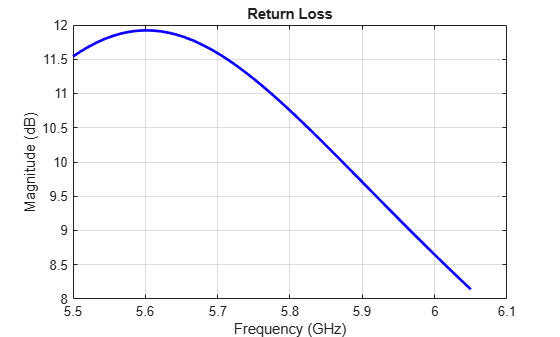### Impedance matching - Adding load at the feed

You can obtain better matching over the entire frequency range by adding some impedance at the feed. As impedance variation is quite smooth a single impedance value might be enough to obtain a good match over the entire frequency range. We select the impedance at 5.8 GHz and try to match it exactly to 50 ohms. A blue dot is added at the feed indicating the location of the lumped load.

```le.Impedance = complex(18, -12); ant.Load = le; figure; show(ant);```Calculating the impedance, indicates that a constant 18 ohms is added to the resistance while a capacitive reactance of 12 ohms is added to the reactance over the entire frequency range. This also changes the resonant frequency of the antenna.

`figure; impedance(ant, freq);`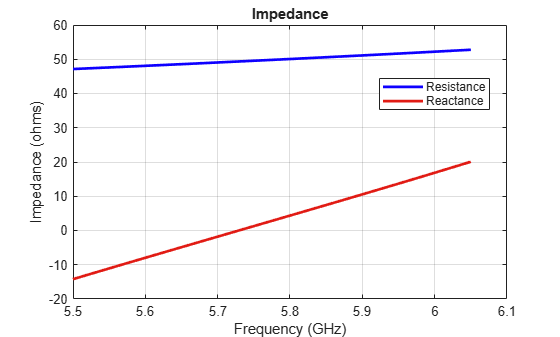However, changing the impedance over the entire frequency range does help with the impedance bandwidth. A value greater than 10 dB of return loss is seen over the entire frequency range of operation.

`figure; returnLoss(ant, freq);`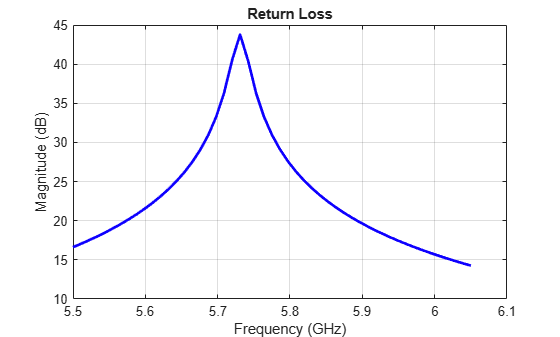### Adding load at arbitrary location on the antenna surface

You can also load the antenna at an arbitrary location on the surface by specifying the x, y and z coordinates of the location. Consider the same cloverleaf antenna but backed by a circular cavity as shown below.

```ref = design(cavityCircular, 5.5e9); ref.Exciter = cloverleaf; figure; show(ref);```You can add some load to the cavity base to add more loss into the system. The blue dot added to the cavity base is the location of the lumped load.

```refload = lumpedElement('Impedance',complex(20, 20), 'Location', [0 10e-3 0]); ref.Load = refload; figure; show(ref);```### Adding multiple loads

Multiple loads can be added to an antenna by specifying multiple lumpedElement. You can specify these either at the feed or on the antenna surface. Multiple blue dots are observed on the antenna surface indicating the location of the load.

```refload2 = lumpedElement('Impedance', complex(30, -10), 'Location', [10e-3, 10e-3, 0]); ref.Load = [refload, refload2]; figure; show(ref);```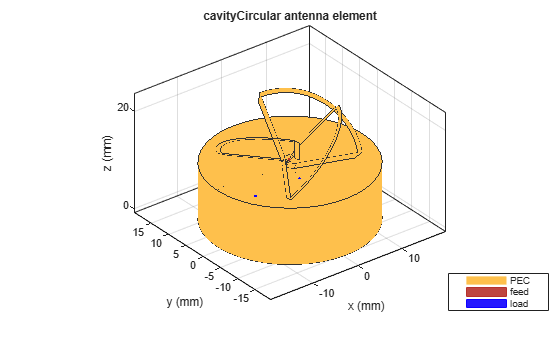All the analysis performed on the above antenna will take into account the effect of the lumped load. This is done by adding the impedance value specified in the lumped load to the basis function in the interaction matrix of Method of Moments. The edge at which the load is added can be visualized by looking at the mesh. The common edge shared by the two blue triangles is the edge at which the impedance value gets added.

```z = impedance(ref, 5.5e9); figure; mesh(ref);```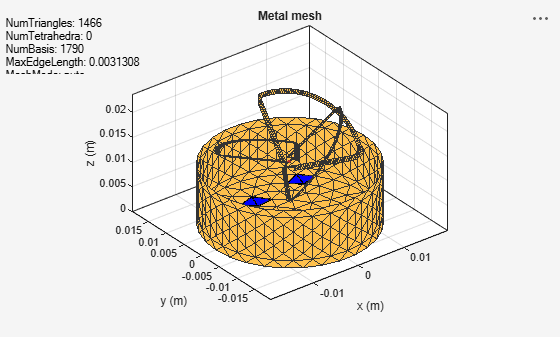## Support

#### Hybrid Beamforming for Massive MIMO Phased Array Systems

Download the white paper